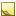﻿How to calculateHow to calculate

The built-in functions and the [Calculated] section of your template let you convert and manipulate values through common operations, concatenation, and if-then-else tests.

This topic contains the following sections:How to assign data types and units

Very commonly in templates, you need to convert raw numbers into Data types and units that TDE can use. You achieve this by specifying data types and units parenthetically after the column name that is receiving the input.Tip

For all Tables you are accessing, always check the Data types of the columns you are using and the supported and default units for all quantifiable data.

In this template, the incoming FuelVolume number is both assigned the datatype of Volume and the source unit of US gallon. The lat/lon numbers are both assigned the datatype of Angle and the source unit of Degree.

```[Template]
TemplateVersion = 1.0
TableID = FuelTransaction-1.0
TemplateName = FuelWithPoints
Intent = Create

[Output]
unitId(VehicleIdMap) = Input.VehicleName
Latitude(Angle,Degree) = Input.Latitude
Longitude(Angle,Degree) = Input.Longitude
FuelVolume(Volume,US gallon) = Input.FuelVolume
...```How to prefix or postfix a value

In this template, concatenation in the [Output] section solves the problem of how to automatically update a driver's Id and Nickname to the same incoming value, yet also postfix the driver's name to the Nickname in the same template run.

```[Template]
TemplateVersion = 1.0
TableID = Driver-1.0
TemplateName = DriverUpdate
Intent = Update

[Output]
Id(AcmeId) = Input.Tag
Nickname = Input.Tag || "_" || Input.FirstName```

So, an input row with a Tag of ABC123 and a FirstName of George would update TDE with these values for the external key and the Nickname, respectively:

`ABC123,ABC123_George`How to use IF THEN ELSE

In this template, the [Calculated] section solves the problem of how to automatically set the correct transaction type based on a separate value (MSGTYPE) that imports with each record. The calculated value, _transactionType, evaluates this rule:

"If the message type equals 1100, then set the transaction type to Auth; otherwise, set it to Sale."

```[Template]
TemplateVersion = 1.0
TableID = FuelTransaction-1.0
TemplateName = FuelWithPoints
Intent = Create

[Calculated]
_transactionType = Input.MSGTYPE == "1100" ? "Auth" : "Sale"

[Output]
TransactionType = _transactionType
unitId(VehicleIdMap) = Input.ASSET_ID
PurchaseTime(Timestamp) = Input.GMT_DATE
...```How to calculate time zones

In this template, the [Calculated] section solves the problem of how to automatically adjust timestamps based on timezone information that is stored simply as numbers. The Calculated values are set to valid TDEData types, and the Timestamp value includes the formatting pattern to be converted.Tip

Note that the time zone mapping used in this input file is explained in a comments section in the template itself; this is a best practice to ensure that others will be able to follow what the template is doing.

```[Template]
TemplateVersion = 1.0
TableID = Timesheet-1.0
TemplateName = TimesheetClockOut
Intent = Create

[Columns]
0, Acme Employee Number
1, TimeIn
2, TimeOut
3, TimeZone

# Time Zone Mapping
# 02 = Hawaii (UTC-10)
# 04 = Pacific (UTC-8)
# 05 = Mountain (UTC-7)
# 06 = Central (UTC-6)
# 07 = Eastern (UTC-5)

[Calculated]
LocalTime(Timestamp, yyyyMMddHHmmss) = Input.TimeOut
TimeZoneNum(Number) = Input.TimeZone

[Output]
DriverId(Acme) = String.PadLeft(Input.[Acme Employee Number], 9, "0")
ShiftOn = "False"
ShiftTime = LocalTime + TimeSpan.FromHours(12 - TimeZoneNum)

[Filter]
TimeOut = GreaterThan(0)```

Notice how the DriverId being imported is specially formatted using a .NET String function, PadLeft. This forces all incoming Ids to be a uniform length (nine digits long), with zeros padding the unused initial positions:

`000987675`How to calculate with timespans

In this template, the [Calculated] section solves the problem of how to automatically adjust incoming timestamps that need an offset correction when being imported to TDE.Tip

TDE supports several .NET system functions, including TimeSpan and DateTime functions. You can use them to add, compare, parse, and convert TimeInterval and Timestamp data.

```[Template]
TemplateVersion = 1.0
TableID = Signature-1.0
TemplateName = TimespanCreateSignature
Intent = Create

[Calculated]